LCM

Common multiple of three numbers is 3276. One number is in this number 63 times, second 7 times, third 9 times. What are the numbers?

Result

a =  52
b =  468
c =  364

Solution:Leave us a comment of this math problem and its solution (i.e. if it is still somewhat unclear...):Be the first to comment!To solve this verbal math problem are needed these knowledge from mathematics:

Do you want to calculate least common multiple two or more numbers?

Next similar math problems:

1. Lesson exercising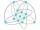The lesson of physical education, pupils are first divided into three groups so that each has the same number. The they redistributed, but into six groups. And again, it was the same number of children in each group. Finally they divided into nine equal gr
2. Three buses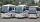Three public transport buses depart together from the bus station in the morning. The first bus was returning to the station after 18 minutes, the second after 12 minutes and a third after 24 minutes. How long will again together on the station? Result exp
3. Buses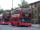At the bus stop is at 10 o'clock met buses No. 2 and No. 9. Bus number 2 runs at an interval of 4 minutes and the bus number 9 at intervals of 9 minutes. How many times the bus meet to 18:00 local time?
4. The smallest numberWhat is the smallest number that can be divided by both 5 and 7
5. LCM of two numberFind the smallest multiple of 63 and 147
6. Lcm simpleFind least common multiple of this two numbers: 140 175.
7. BiketrialKamil was biketrial. Before hill he set the forward gear with 42 teeth and the back with 35 teeth. After how many exercises (rotation) of the front wheel both wheels reach the same position?
8. Apples 2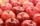How many minimum apples are in the cart, if possible is completely divided into packages of 6, 14 and 21 apples?
9. Dance ensemble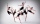The dance ensemble took the stage in pairs. During dancing, the dancers gradually formed groups of four, six and nine. How many dancers have an ensemble?
10. Balls groups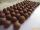Karel pulled the balls out of his pocket and divide it into the groups. He could divide them in four, six or seven, and no ball ever left. How little could be a ball?
11. CherriesCherries in the bowl can be divided equally among 19 or 13 or 28 children. How many is the minimum cherries in the bowl?
12. Multiples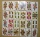What is the sum of the multiples of number 7 that are greater than 30 but less than 56?
13. Addition of Roman numbersAdded together and write as decimal number: LXVII + MLXIV
14. Primes 2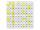Which prime numbers is number 2025 divisible?
15. NumberDetermine unknown number if you know that difference between five times and triple of number is 42.
16. If-then equationIf 5x - 17 = -x + 7, then x =
17. Line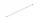How many parts of line divide 5 (different) points that lie on it?